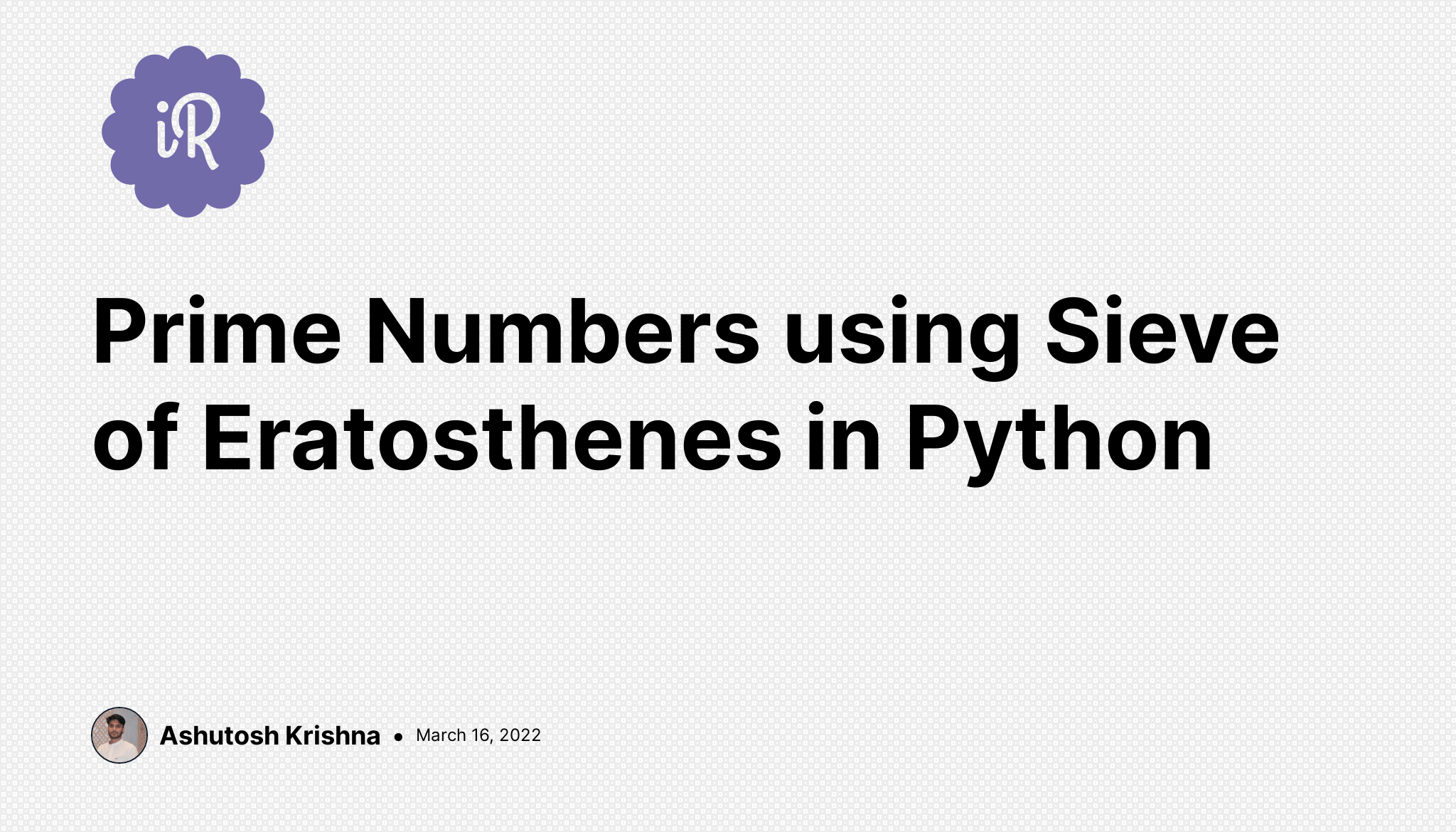# Ashutosh Writes# Prime Numbers using Sieve of Eratosthenes in Python

A prime number, as we know, is a natural number that has exactly two distinct natural number divisors: the number 1 and itself.

We often get questions like the one given below:

Given a number n, print all primes smaller than or equal to n. — e.g. If n is 20, the output should be “2, 3, 5, 7, 11, 13, 17, 19”

Sieve of Eratosthenes is used to get all prime numbers in a given range and is a very efficient algorithm. You can read more about Sieve of Eratosthenes on Wikipedia.

In this algorithm, we follow the following steps to get prime numbers up to n (let’s suppose n = 12):

1. First, we create a boolean list of length n+1 filled with all True as:\ `is_prime = [True, True, True, True, True, True, True, True, True, True, True, True, True]`
2. Since, 0 and 1 are not primes, we make the first two elements of the list as False. So, now our list is:\ `is_prime = [False, False, True, True, True, True, True, True, True, True, True, True, True]`
3. Starting from 2, we make elements at index that are multiples of 2 as False, except itself. So, our list becomes:\ `is_prime = [False, False, True, True, False, True, False, True, False, True, False, True, False]`
4. Repeat the step 3 till square root of n (i.e. √12 in this example). At the end, our list becomes :\ `is_prime = [False, False, True, True, False, True, False, True, False, False, False, True, False]`
5. The remaining list contains True only at the index which is prime.
``````def seive_of_eratosthenes(n):
is_prime = [True for _ in range(n+1)]
is_prime, is_prime = False, False

for i in range(2, int(n**0.5)+1):
for j in range(2*i, n+1, i):
is_prime[j] = False

return is_prime

if __name__ == "__main__":
n = int(input("Enter the number upto which : "))
li = seive_of_eratosthenes(n)
for i in range(n+1):
if li[i]:
print(i, end=" ")
``````

This code is a straightforward representation of the steps mentioned above.

We first make a boolean list with all values initialized with True. A value will be False if it is not prime else True. Then we start iterating from 2 to the square root of the number and updating the multiples as False as well. This is done using another ‘for’ loop from 2*i to n+1 with a step of j+1.

In the end, we get our final list and check the values. If the value at any index is True, it means the number is prime and we print the number.